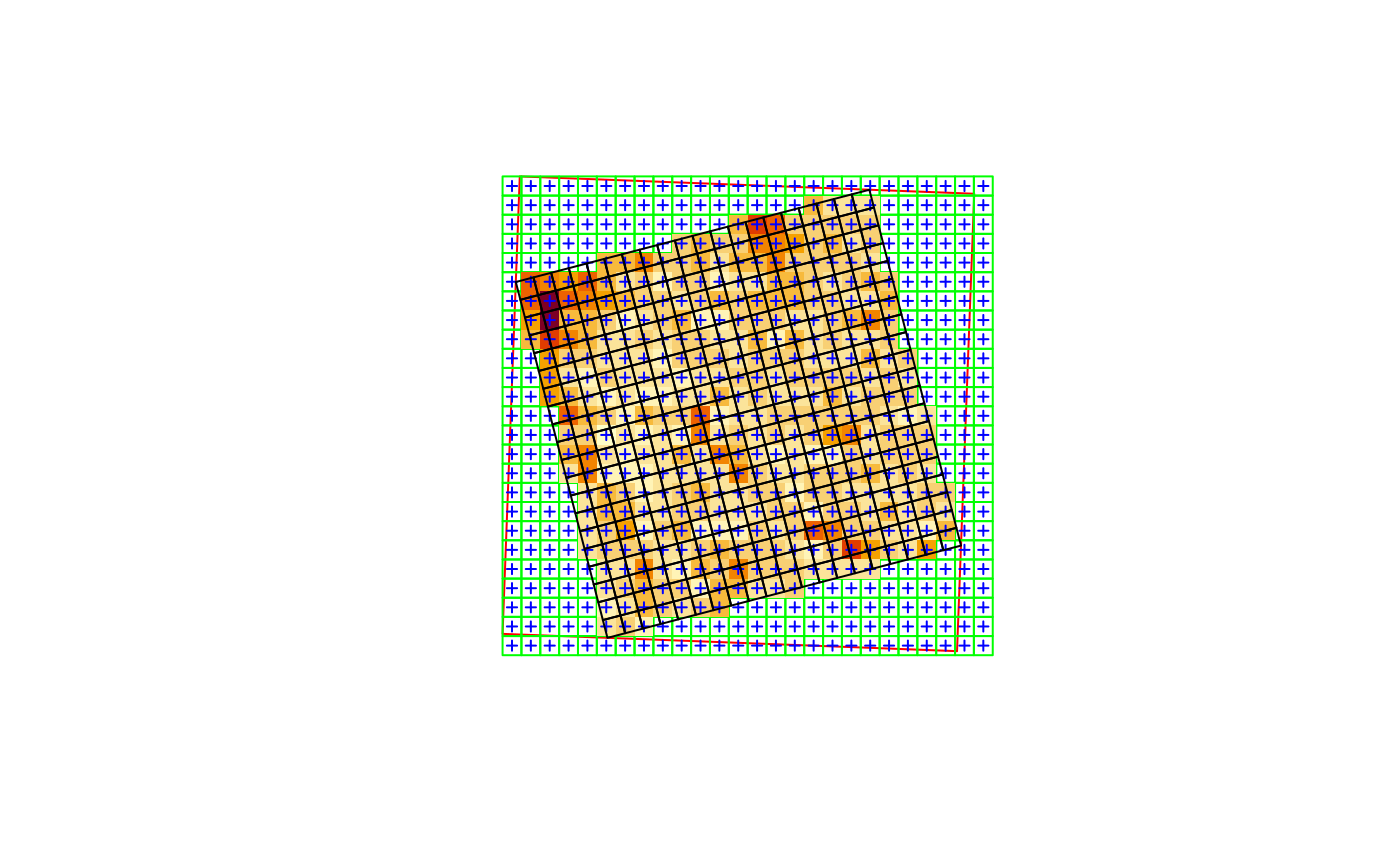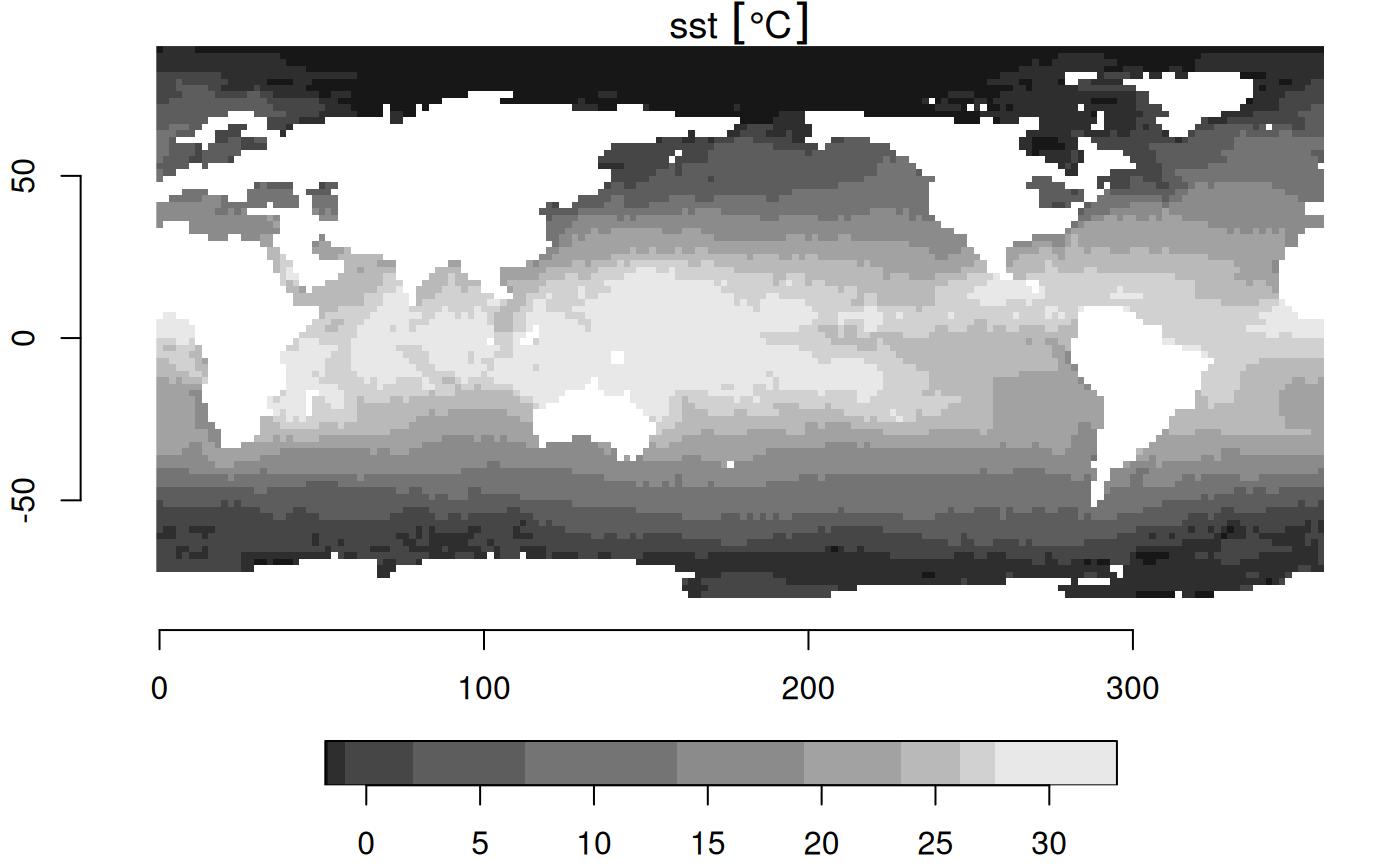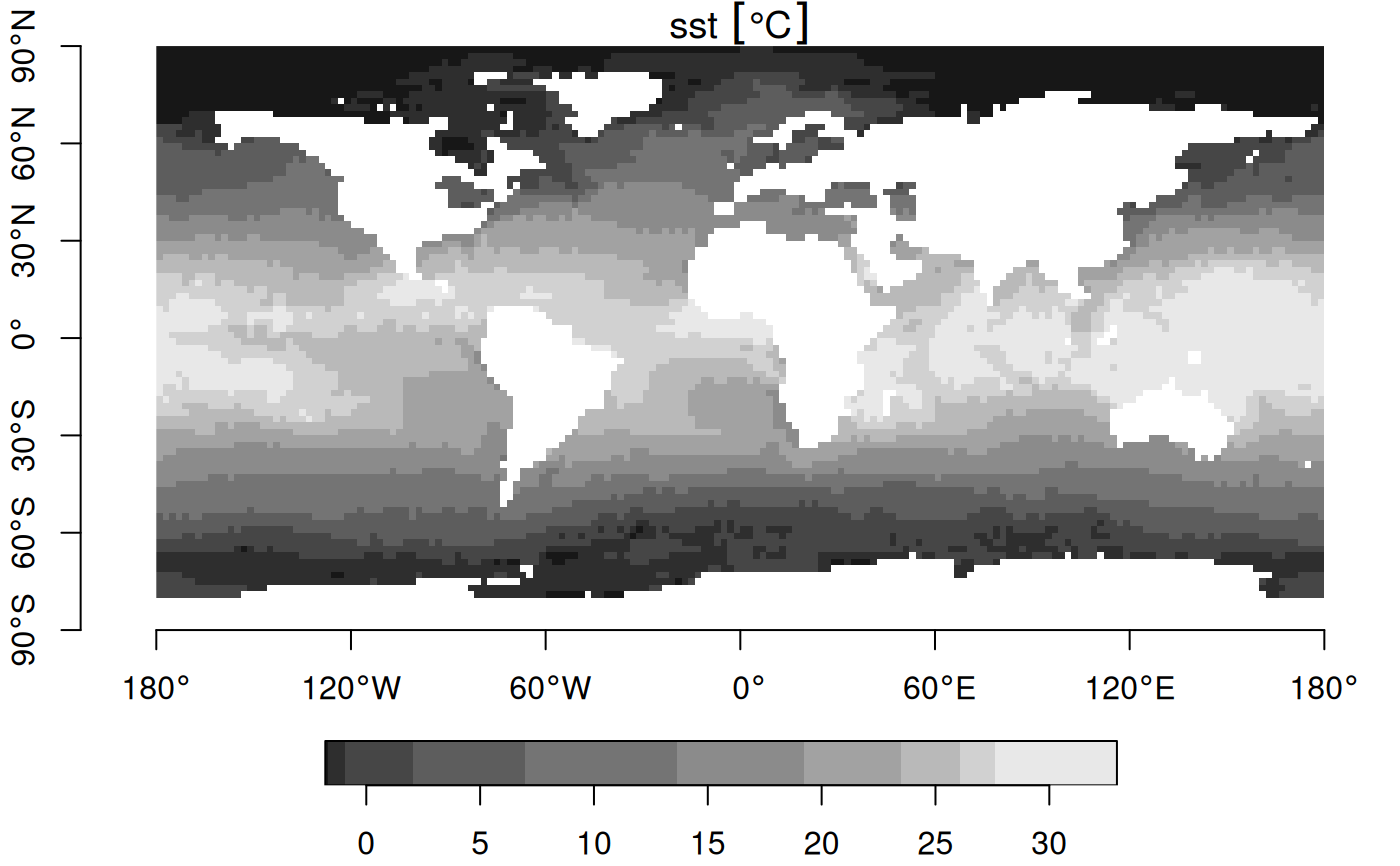Warp (resample) grids in stars objects to a new grid, possibly in an new coordinate reference system

## Usage

st_warp(
src,
dest,
...,
crs = NA_crs_,
cellsize = NA_real_,
segments = 100,
use_gdal = FALSE,
options = character(0),
no_data_value = NA_real_,
debug = FALSE,
method = "near",
threshold = NA_real_
)

## Arguments

src

object of class stars with source raster

dest

object of class stars with target raster geometry

...

ignored

crs

coordinate reference system for destination grid, only used when dest is missing

cellsize

length 1 or 2 numeric; cellsize in target coordinate reference system units

segments

(total) number of segments for segmentizing the bounding box before transforming to the new crs

use_gdal

logical; if TRUE, use gdal's warp or warper, through gdal_utils

options

character vector with options, passed on to gdalwarp

no_data_value

value used by gdalwarp for no_data (NA) when writing to temporary file; not setting this when use_gdal is TRUE leads to a warning

debug

logical; if TRUE, do not remove the temporary gdalwarp destination file, and print its name

method

character; see details for options; methods other than near only work when use_gdal=TRUE

threshold

numeric; distance threshold for warping curvilinear grids: new cells at distances larger than threshold are assigned NA values.

## Details

method should be one of near, bilinear, cubic, cubicspline, lanczos, average, mode, max, min, med, q1 or q3; see https://github.com/r-spatial/stars/issues/109

For gridded spatial data (dimensions x and y), see figure; the existing grid is transformed into a regular grid defined by dest, possibly in a new coordinate reference system. If dest is not specified, but crs is, the procedure used to choose a target grid is similar to that of projectRaster. This entails: (i) the envelope (bounding box polygon) is transformed into the new crs, possibly after segmentation (red box); (ii) a grid is formed in this new crs, touching the transformed envelope on its East and North side, with (if cellsize is not given) a cellsize similar to the cell size of src, with an extent that at least covers x; (iii) for each cell center of this new grid, the matching grid cell of x is used; if there is no match, an NA value is used.

## Examples

geomatrix = system.file("tif/geomatrix.tif", package = "stars")
#> stars object with 2 dimensions and 1 attribute
#> attribute(s):
#>                Min. 1st Qu. Median    Mean 3rd Qu. Max.
#> geomatrix.tif    74     107    123 126.765     132  255
#> dimension(s):
#>   from to  offset delta                refsys point x/y
#> x    1 20 1841002   1.5 WGS 84 / UTM zone 11N  TRUE [x]
#> y    1 20 1144003  -1.5 WGS 84 / UTM zone 11N  TRUE [y]
#> sheared raster with parameters: -5 -5
new_crs = st_crs('OGC:CRS84')
y = st_warp(x, crs = new_crs)
plot(st_transform(st_as_sfc(st_bbox(x)), new_crs), col = NA, border = 'red')
plot(st_as_sfc(y, as_points=FALSE), col = NA, border = 'green', axes = TRUE, add = TRUE)
image(y, add = TRUE, nbreaks = 6)
plot(st_as_sfc(y, as_points=TRUE), pch=3, cex=.5, col = 'blue', add = TRUE)
plot(st_transform(st_as_sfc(x, as_points=FALSE), new_crs), add = TRUE)# warp 0-360 raster to -180-180 raster:
r = read_stars(system.file("nc/reduced.nc", package = "stars"))
#> sst, anom, err, ice,
r %>% st_set_crs('OGC:CRS84') %>% st_warp(st_as_stars(st_bbox(), dx = 2)) -> s
plot(r, axes = TRUE) # no CRS set, so no degree symbols in labelsplot(s, axes = TRUE)# downsample raster (90 to 270 m)
r = read_stars(system.file("tif/olinda_dem_utm25s.tif", package = "stars"))
r270 = st_as_stars(st_bbox(r), dx = 270)
r270 = st_warp(r, r270)